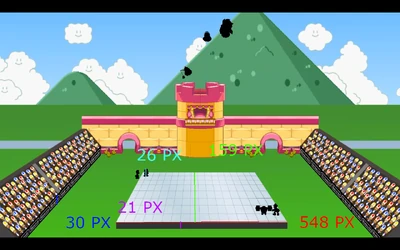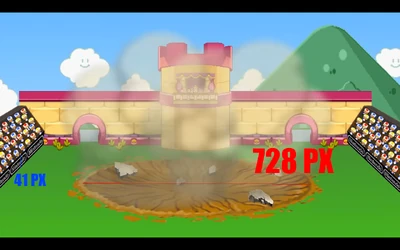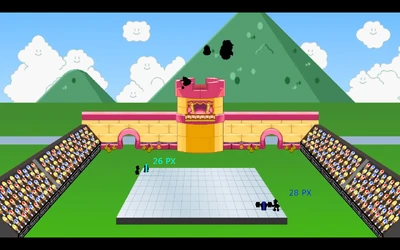4,251 Pages

The SMBZ reboot characters have been listed as Unknown for the longest time. And I'm here to hopefully change that

## Big Kaboom

### Stage Destruction End

A large Bob-Omb is dropped onto the arena and Mario, Luigi, Wario, and Waluigi survive being at its epicenter completely unscathed. Now there aren't many good shots to compare the characters to the crater made (That I'm aware of) so I'll just find the value for destroying the large platform Mario was on.

Using Mario's height of 5' 1" (154.94 Centimeters) = 26 Pixels = 5.95923076923 cm/pixelArena length = 548 pixels = 3,265.65846154 Centimeters

Arena Width = 159 pixels = 947.517692308 Centimeters

Arena Height = 21 pixels = 125.143846154 Centimeters

Stand Height (For something else) = 30 pixels = 178.776923077 Centimeters

Arena Volume = 3,265.65846154 * 947.517692308 * 125.143846154 = 387,228,744.888 Cubic Centimeters

Seeing as most of the arena was completely destroyed with just a few chunks remaining, violent fragmentation seems likely

Violent Fragmentation of Concrete is 20 joules/cc^3. So

387,228,744.888 * 20 = 7,744,574,897.76 joules, or 1.85099782451243 Tons of TNT. Building level+

And as Mario and Co. tank this with no damage and they're able to harm each other, this scales to everyone.

### Explosion Size EndFound a shot to get the explosion's size, so I'll see what results this gets.

Stand Height = 178.776923077 Centimeters = 41 pixels = 4.36041275798 cm/px

Crater/Explosion length = 728 pixels = 31.7438048781 Meters

Explosion formula = R^3*((27136*P+8649)^(1/2)/13568-93/13568)^2

15.8719024391^3*((27136*1.37895+8649)^(1/2)/13568-93/13568)^2 = 0.32134568393 Tons of TNT, still Building level, but nearly baseline

## Wario is StronkMario's height = 154.94 Centimeters = 26 Pixels

Wario's Height = 28 Pixels = 166.858461538 CentimetersWario's Height = 166.858461538 Centimeters = 324 Pixels = 0.51499525166 Cm/Pixel

Bar Radius = 25/2 Pixels = 12.5 Pixels = 6.43744064576 Centimeters

Bar Length = 495 Pixels = 254.922649572 Centimeters

Bar Volume = π * 6.43744064576^2 * 254.922649572 = 33,188.3 Cm^3

Weights Radius = 162/2 Pixels = 81 Pixels = 41.7146153845 Centimeters

Weights Length = 108 Pixels = 55.6194871793 Centimeters

Weights Volume = π * 41.7146153845^2 * 55.6194871793 = 304,055.833698 Cm^3

Now thats the volume of solid metal, but these weights have empty space in the middle for the bar

Smaller Bar Volume = π * 6.43744064576^2 * 55.6194871793 = 7,241.07971581 Cm^3

304,055.833698 - 7,241.07971581 = 296,814.753982 Cm^3 * 2 (As there's 2 of these) = 593,629.507964 Cm^3

These kinds of weights are usually made of Steel (With a density of 7,850 Kilograms/Meter^3)

(0.593629507964 + 0.304055833698) * 7,850 = 7,046.82993205 Kilograms, Class 10

## Professor Elvin Gadd’s car is fest as frick

E. Gadd’s Poltergust drives/flies 240 Miles (386.243 Kilometers) in roughly 8.5 seconds

386,243/8.5 = 45,440.3529412 Meters/Second = Mach 132.479 Massively Hypersonic

Shadow could dodge this thing flying at him, so this scales to everyone’s reaction speed

Community content is available under CC-BY-SA unless otherwise noted.